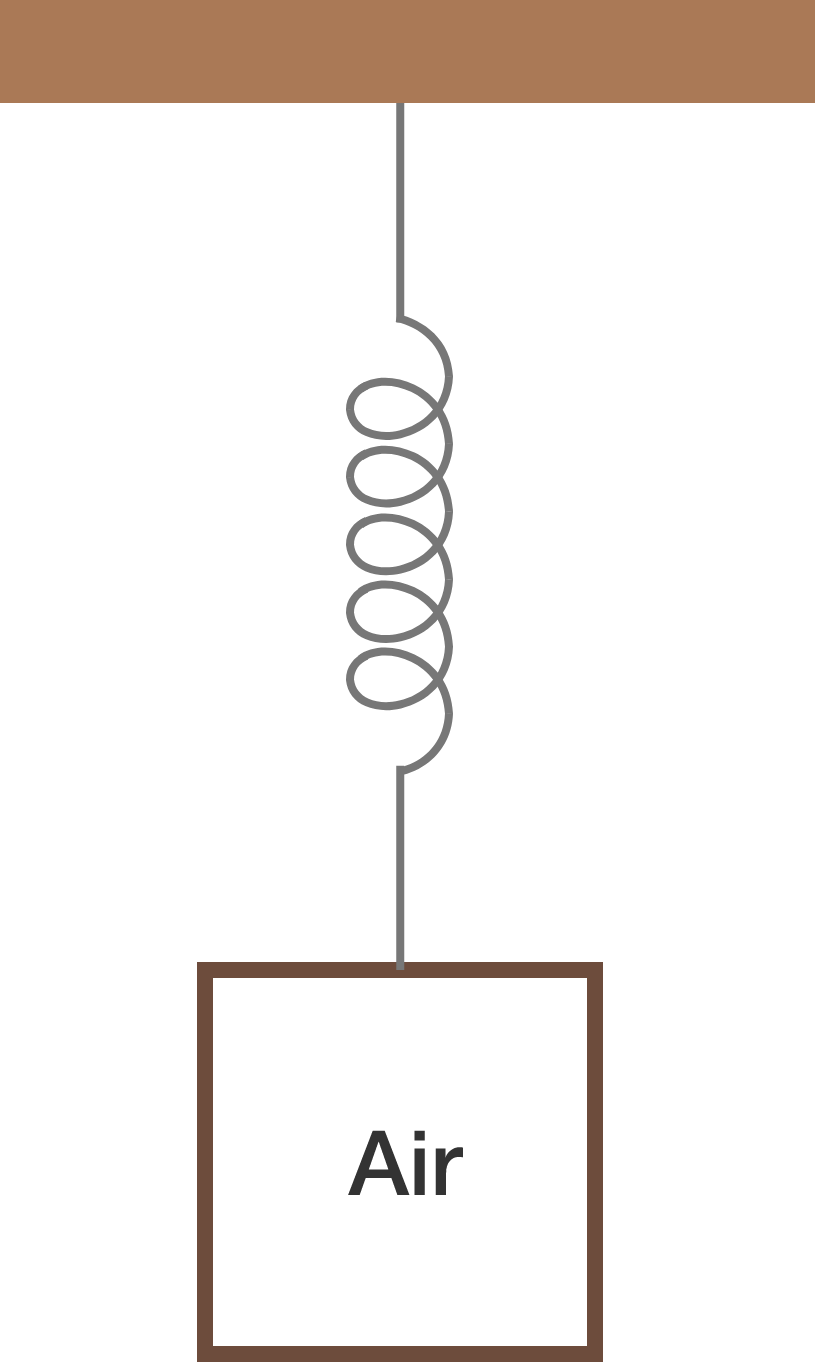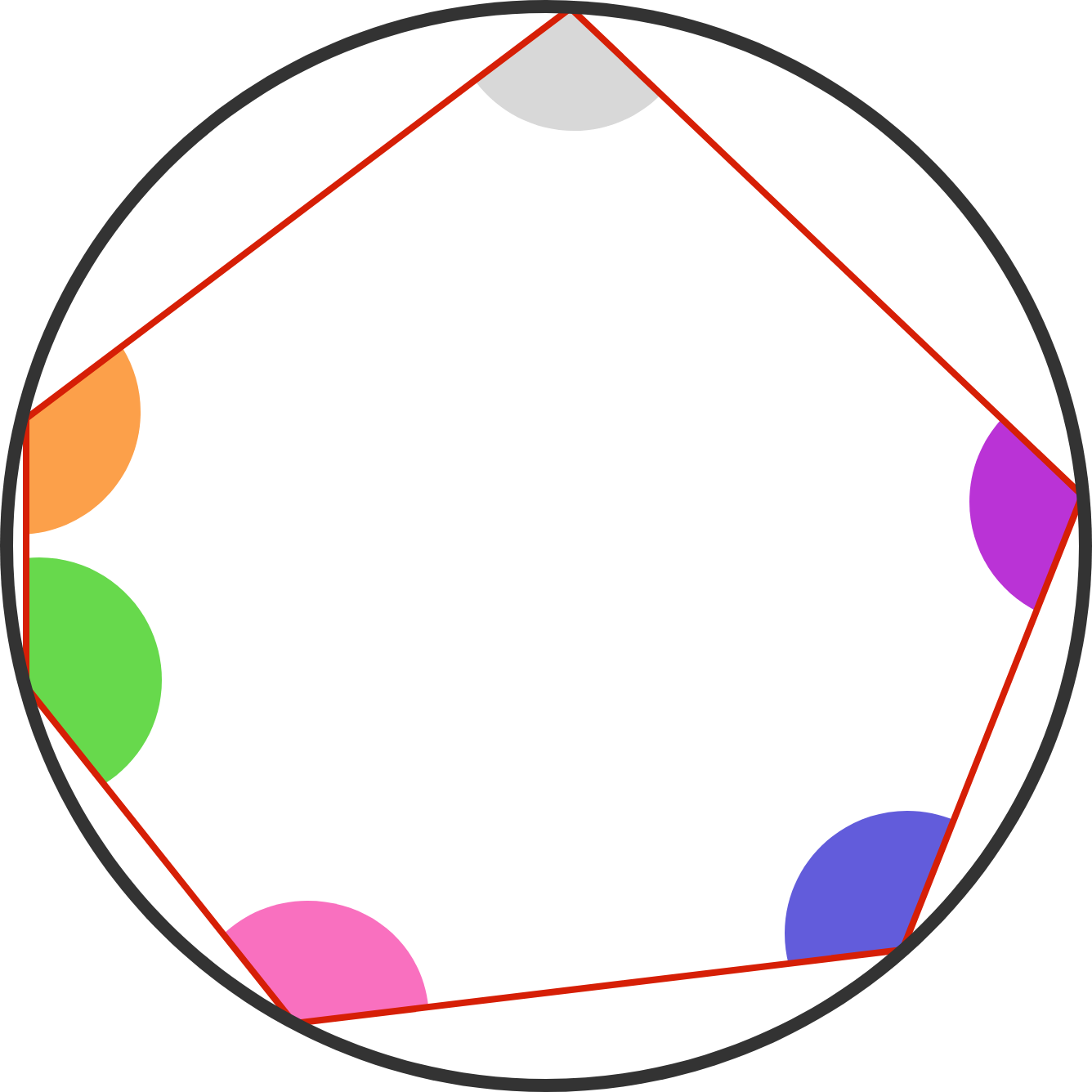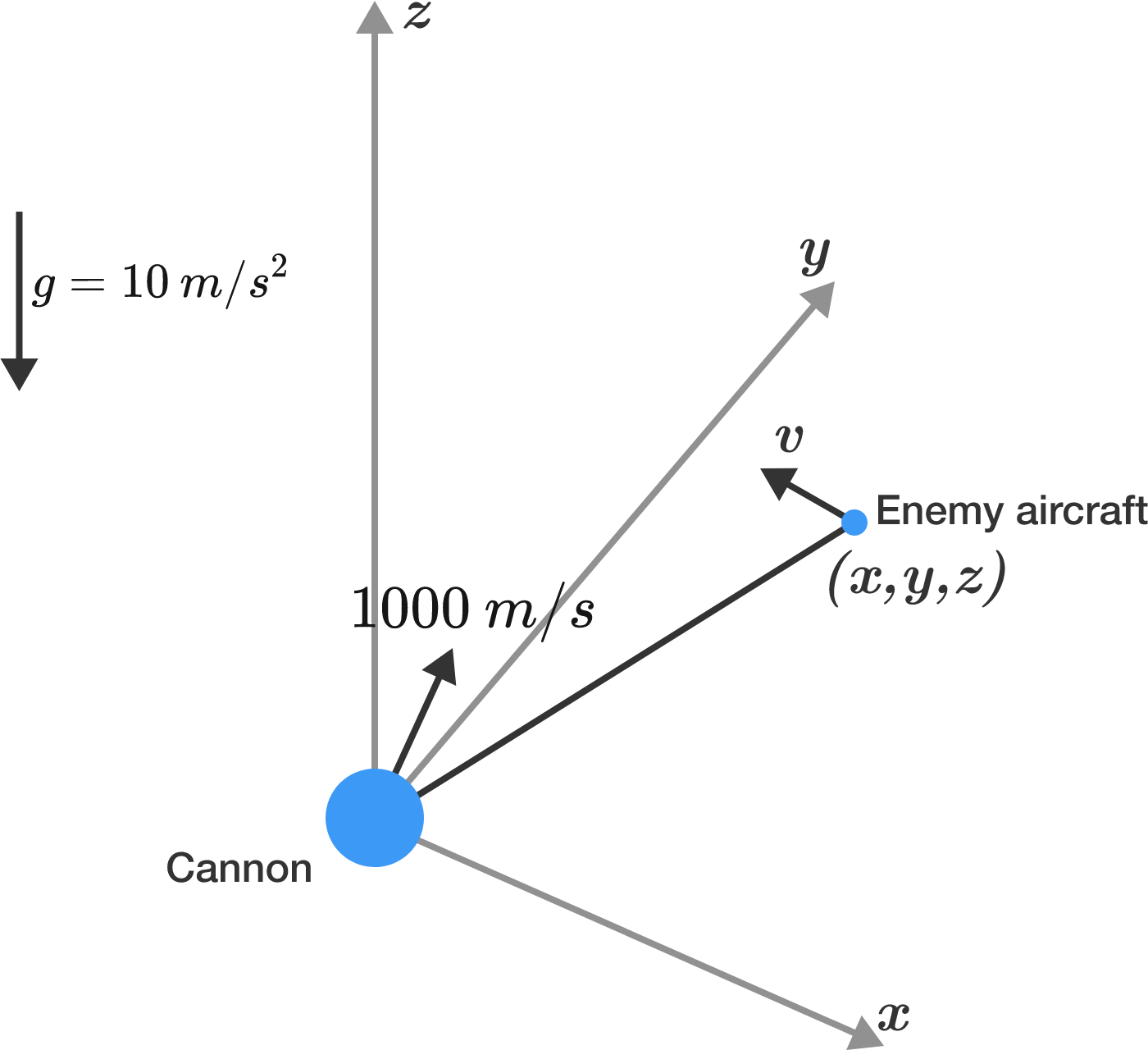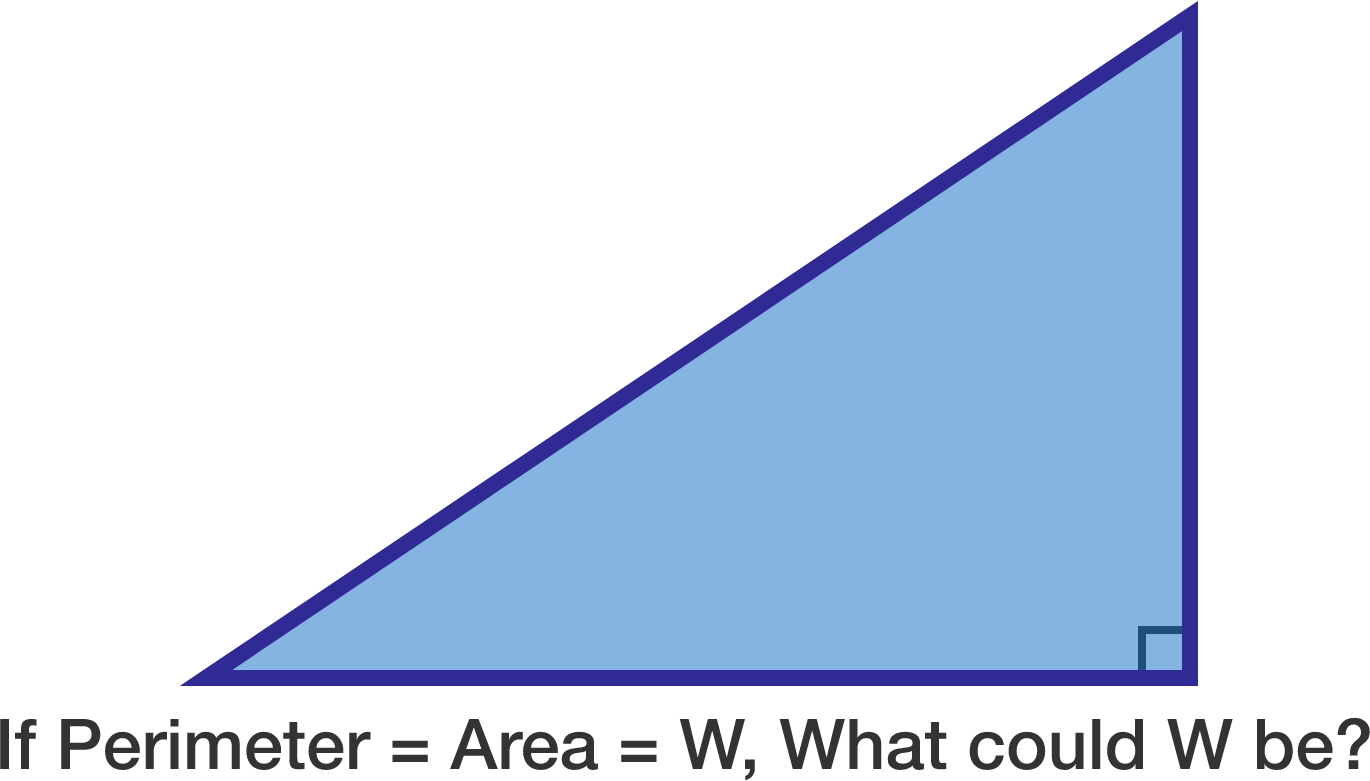# Problems of the Week

Contribute a problem

# 2017-07-17 IntermediateA hollow, metal box is filled with gas and hung from the ceiling of a laboratory by a perfectly elastic spring. Furthermore, the laboratory is held under a perfect vacuum.

At time zero, you pull the box down and let it go, setting it to oscillate. What will happen to the box after a long time?Does there exist a hexagon whose 6 vertices lie on a circle, and whose internal angles are $70^\circ, 90^\circ, 110^\circ, 130^\circ, 150^\circ, 170^\circ$ in some order?

You are a soldier, and your base is under attack by enemy aircraft. At time $t = 0$, one such aircraft is positioned at $(x,y,z) = (1000\text{ m, }2000 \text{ m, }3000 \text{ m}).$ The aircraft has a constant velocity of $(v_x,v_y,v_z) = (-400 \text{ m/s, }+400 \text{ m/s, }+400 \text{ m/s}).$

Also at $t = 0,$ you take control of an anti-aircraft artillery cannon and fire a shot to bring down the enemy plane. The cannon has a muzzle velocity of $1000 \text{ m/s},$ and it can be aimed in any direction. Your position is $(x,y,z) = (0 \text{ m, }0 \text{ m, }0\text{ m}).$There is an ambient gravitational acceleration of $10 \text{ m/s}^2$ in the $-z$ direction (downward).

At what time (in seconds) does the artillery shell strike the enemy plane?


Details and Assumptions:

• Neglect air resistance.
• From a targeting perspective, consider the enemy plane to be a point-particle.
• You are a superlative marksman.
• If you find more than one solution, give the smaller value (provided that it's not negative).

$p, q,$ and $(p^q+q^p)$ are all prime numbers. What is the largest possible sum of the three numbers: $p+q+(p^q+q^p) ?$

Find all the right triangles with integer side lengths such that their perimeter and area have the same numerical value.

Submit your answer as the sum of all possible numerical values mentioned above.×